Mathematics
Easy

Question

# Simplify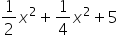##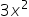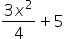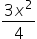5Hint:

## The correct answer is:### The given expression is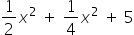There is one variable in the above expression. The variable is: ‘x’. There are three terms in the above expression. We have to perform the operations between them and simplify the expression.If we see, first and second term contains the same variable raised to same power 2. Such terms are called “like terms.” And the terms with different variables are called “unlike terms.”The third term is a constant.For like terms, we can perform the operations between their coefficients by keeping the variable constant. It means we can treat each term as a constant and perform operations like normal operations on constants.For the above expression, we can perform an operation between the first term and second term as both the terms as they have the same variable ‘x2’. We will keep ‘x2 ' constant and perform addition between the coefficients.Let’s see the expression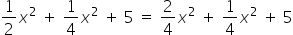To make the denominators same of the first term we multiplied and divided the first term by 2Solving further we get,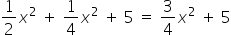Therefore, the value of the equation is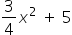We have to be careful about the powers of the variables too. If the variable is same and power is different, we cannot perform the operation any further.

### Related Questions to study#### With Turito Foundation.#### Get an Expert Advice From Turito.